Eric Smith

Slide Duration:

Section 1: Properties of Real Numbers
Basic Types of Numbers

30m 41s

Intro
0:00
Objectives
0:07
Basic Types of Numbers
0:36
Natural Numbers
1:02
Whole Numbers
1:29
Integers
2:04
Rational Numbers
2:38
Irrational Numbers
5:06
Imaginary Numbers
6:48
Basic Types of Numbers Cont.
8:09
The Big Picture
8:10
Real vs. Imaginary Numbers
8:30
Rational vs. Irrational Numbers
8:48
Basic Types of Numbers Cont.
10:55
Number Line
11:06
Absolute Value
11:44
Inequalities
12:39
Example 1
13:16
Example 2
17:30
Example 3
21:56
Example 4
24:27
Example 5
27:48
Operations on Numbers

19m 26s

Intro
0:00
Objectives
0:06
Operations on Numbers
0:25
0:53
Subtraction
1:33
Multiplication & Division
2:19
Exponents
3:24
Bases
4:04
Square Roots
4:59
Principle Square Roots
5:09
Perfect Squares
6:32
Simplifying and Combining Roots
6:52
Example 1
8:16
Example 2
12:30
Example 3
14:02
Example 4
16:27
Order of Operations

12m 6s

Intro
0:00
Objectives
0:06
The Order of Operations
0:25
Work Inside Parentheses
0:42
Simplify Exponents
0:52
Multiplication & Division from Left to Right
0:57
Addition & Subtraction from Left to Right
1:11
Remember PEMDAS
1:21
The Order of Operations Cont.
2:27
Example
2:43
Example 1
3:55
Example 2
5:36
Example 3
7:35
Example 4
8:56
Properties of Real Numbers

18m 52s

Intro
0:00
Objectives
0:07
The Properties of Real Numbers
0:23
Commutative Property of Addition and Multiplication
0:44
Associative Property of Addition and Multiplication
1:50
Distributive Property of Multiplication Over Addition
3:20
Division Property of Zero
4:46
Division Property of One
5:23
Multiplication Property of Zero
5:56
Multiplication Property of One
6:17
6:29
Why Are These Properties Important?
6:53
Example 1
9:16
Example 2
13:04
Example 3
14:30
Example 4
16:57
Section 2: Linear Equations
The Vocabulary of Linear Equations

12m 22s

Intro
0:00
Objectives
0:09
The Vocabulary of Linear Equations
0:44
Variables
0:52
Terms
1:09
Coefficients
1:40
Like Terms
2:18
Examples of Like Terms
2:37
Expressions
4:01
Equations
4:26
Linear Equations
5:04
Solutions
5:55
Example 1
6:16
Example 2
7:16
Example 3
8:45
Example 4
10:20
Solving Linear Equations in One Variable

28m 52s

Intro
0:00
Objectives
0:08
Solving Linear Equations in One Variable
0:34
Conditional Cases
0:51
Identity Cases
1:09
1:30
Solving Linear Equations in One Variable Cont.
2:00
2:10
Multiplication Property of Equality
2:43
Steps to Solve Linear Equations
3:14
Example 1
4:22
Example 2
8:21
Example 3
12:32
Example 4
14:19
Example 5
17:25
Example 6
22:17
Solving Formulas

12m 2s

Intro
0:00
Objectives
0:06
Solving Formulas
0:18
Formulas
0:26
Use the Same Properties as Solving Linear Equations
1:36
1:55
Multiplication Property of Equality
1:58
Steps to Solve Formulas
2:43
Example 1
3:56
Example 2
6:09
Example 3
8:39
Applications of Linear Equations

28m 41s

Intro
0:00
Objectives
0:10
Applications of Linear Equations
0:43
The Six-Step Method to Solving Word Problems
0:55
Common Terms
3:12
Example 1
5:03
Example 2
9:40
Example 3
13:48
Example 4
17:58
Example 5
23:28
Applications of Linear Equations, Motion & Mixtures

24m 26s

Intro
0:00
Objectives
0:21
Motion and Mixtures
0:46
Motion Problems: Distance, Rate, and Time
1:06
Mixture Problems: Amount, Percent, and Total
1:27
The Table Method
1:58
The Beaker Method
3:38
Example 1
5:05
Example 2
9:44
Example 3
14:20
Example 4
19:13
Section 3: Graphing
Rectangular Coordinate System

22m 55s

Intro
0:00
Objectives
0:11
The Rectangular Coordinate System
0:39
The Cartesian Coordinate System
0:40
X-Axis
0:54
Y-Axis
1:04
Origin
1:11
1:26
Ordered Pairs
2:10
Example 1
2:55
The Rectangular Coordinate System Cont.
6:09
X-Intercept
6:45
Y-Intercept
6:55
Relation of X-Values and Y-Values
7:30
Example 2
11:03
Example 3
12:13
Example 4
14:10
Example 5
18:38
Slope & Graphing

27m 58s

Intro
0:00
Objectives
0:11
Slope and Graphing
0:48
Standard Form
1:14
Example 1
2:24
Slope and Graphing Cont.
4:58
Slope, m
5:07
Slope is Rise over Run
6:11
Don't Mix Up the Coordinates
8:20
Example 2
9:39
Slope and Graphing Cont.
14:26
Slope-Intercept Form
14:34
Example 3
16:55
Example 4
18:00
Slope and Graphing Cont.
19:00
Rewriting an Equation in Slope-Intercept Form
19:39
Rewriting an Equation in Standard Form
20:09
Slopes of Vertical & Horizontal Lines
20:56
Example 5
22:49
Example 6
24:09
Example 7
25:59
Example 8
26:57
Linear Equations in Two Variables

20m 36s

Intro
0:00
Objectives
0:13
Linear Equations in Two Variables
0:36
Point-Slope Form
1:07
Substitute in the Point and the Slope
2:21
Parallel Lines: Two Lines with the Same Slope
4:05
Perpendicular Lines: Slopes are Negative Reciprocals of Each Other
4:39
Perpendicular Lines: Product of Slopes is -1
5:24
Example 1
6:02
Example 2
7:50
Example 3
10:49
Example 4
13:26
Example 5
15:30
Example 6
17:43
Section 4: Functions
Introduction to Functions

21m 24s

Intro
0:00
Objectives
0:07
Introduction to Functions
0:58
Relations
1:03
Functions
1:37
Independent Variables
2:00
Dependent Variables
2:11
Function Notation
2:21
Function
3:43
Input and Output
3:53
Introduction to Functions Cont.
4:45
Domain
4:46
Range
4:55
Functions Represented by a Diagram
6:41
Natural Domain
9:11
Evaluating Functions
12:02
Example 1
13:13
Example 2
15:03
Example 3
16:18
Example 4
19:54
Graphing Functions

16m 12s

Intro
0:00
Objectives
0:09
Graphing Functions
0:54
Using Slope-Intercept Form
1:56
Vertical Line Test
2:58
Determining the Domain
4:20
Determining the Range
5:43
Example 1
6:06
Example 2
7:18
Example 3
8:31
Example 4
11:04
Section 5: Systems of Linear Equations
Systems of Linear Equations

25m 54s

Intro
0:00
Objectives
0:13
Systems of Linear Equations
0:46
System of Equations
0:51
System of Linear Equations
1:15
Solutions
1:35
Points as Solutions
1:53
Finding Solutions Graphically
5:13
Example 1
6:37
Example 2
12:07
Systems of Linear Equations Cont.
17:01
One Solution, No Solution, or Infinite Solutions
17:10
Example 3
18:31
Example 4
22:37
Solving a System Using Substitution

20m 1s

Intro
0:00
Objectives
0:09
Solving a System Using Substitution
0:32
Substitution Method
1:24
Substitution Example
2:35
One Solution, No Solution, or Infinite Solutions
7:50
Example 1
9:45
Example 2
12:48
Example 3
15:01
Example 4
17:30
Solving a System Using Elimination

19m 40s

Intro
0:00
Objectives
0:09
Solving a System Using Elimination
0:27
Elimination Method
0:42
Elimination Example
2:01
One Solution, No Solution, or Infinite Solutions
7:05
Example 1
8:53
Example 2
11:46
Example 3
15:37
Example 4
17:45
Applications of Systems of Equations

24m 34s

Intro
0:00
Objectives
0:12
Applications of Systems of Equations
0:30
Word Problems
1:31
Example 1
2:17
Example 2
7:55
Example 3
13:07
Example 4
17:15
Section 6: Inequalities
Solving Linear Inequalities in One Variable

17m 13s

Intro
0:00
Objectives
0:08
Solving Linear Inequalities in One Variable
0:37
Inequality Expressions
0:46
Linear Inequality Solution Notations
3:40
Inequalities
3:51
Interval Notation
4:04
Number Lines
4:43
Set Builder Notation
5:24
Use Same Techniques as Solving Equations
6:59
'Flip' the Sign when Multiplying or Dividing by a Negative Number
7:12
'Flip' Example
7:50
Example 1
8:54
Example 2
11:40
Example 3
14:01
Compound Inequalities

16m 13s

Intro
0:00
Objectives
0:07
Compound Inequalities
0:37
'And' vs. 'Or'
0:44
'And'
3:24
'Or'
3:35
'And' Symbol, or Intersection
3:51
'Or' Symbol, or Union
4:13
Inequalities
4:41
Example 1
6:22
Example 2
9:30
Example 3
11:27
Example 4
13:49
Solving Equations with Absolute Values

14m 12s

Intro
0:00
Objectives
0:08
Solve Equations with Absolute Values
0:18
Solve Equations with Absolute Values Cont.
1:11
Steps to Solving Equations with Absolute Values
2:21
Example 1
3:23
Example 2
6:34
Example 3
10:12
Inequalities with Absolute Values

17m 7s

Intro
0:00
Objectives
0:07
Inequalities with Absolute Values
0:23
Recall…
2:08
Example 1
3:39
Example 2
6:06
Example 3
8:14
Example 4
10:29
Example 5
13:29
Graphing Inequalities in Two Variables

15m 33s

Intro
0:00
Objectives
0:07
Graphing Inequalities in Two Variables
0:32
Split Graph into Two Regions
1:53
Graphing Inequalities
5:44
Test Points
6:20
Example 1
7:11
Example 2
10:17
Example 3
13:06
Systems of Inequalities

21m 13s

Intro
0:00
Objectives
0:08
Systems of Inequalities
0:24
Test Points
1:10
Steps to Solve Systems of Inequalities
1:25
Example 1
2:23
Example 2
7:28
Example 3
12:51
Section 7: Polynomials
Integer Exponents

44m 51s

Intro
0:00
Objectives
0:09
Integer Exponents
0:42
Exponents 'Package' Multiplication
1:25
Example 1
2:00
Example 2
3:13
Integer Exponents Cont.
4:50
Product Rule for Exponents
4:51
Example 3
7:16
Example 4
10:15
Integer Exponents Cont.
13:13
Power Rule for Exponents
13:14
Power Rule with Multiplication and Division
15:33
Example 5
16:18
Integer Exponents Cont.
20:04
Example 6
20:41
Integer Exponents Cont.
25:52
Zero Exponent Rule
25:53
Quotient Rule
28:24
Negative Exponents
30:14
Negative Exponent Rule
32:27
Example 7
34:05
Example 8
36:15
Example 9
39:33
Example 10
43:16

18m 33s

Intro
0:00
Objectives
0:07
0:25
Terms
0:33
Coefficients
0:51
1:13
Like Terms
1:29
Polynomials
2:21
Monomials, Binomials, Trinomials, and Polynomials
5:41
Degrees
7:00
Evaluating Polynomials
8:12
9:25
Example 1
11:48
Example 2
13:00
Example 3
14:41
Example 4
16:15
Multiplying Polynomials

25m 7s

Intro
0:00
Objectives
0:06
Multiplying Polynomials
0:41
Distributive Property
1:00
Example 1
2:49
Multiplying Polynomials Cont.
8:22
Organize Terms with a Table
8:23
Example 2
13:40
Multiplying Polynomials Cont.
16:33
Multiplying Binomials with FOIL
16:48
Example 3
18:49
Example 4
20:04
Example 5
21:42
Dividing Polynomials

44m 56s

Intro
0:00
Objectives
0:07
Dividing Polynomials
0:29
Dividing Polynomials by Monomials
2:10
Dividing Polynomials by Polynomials
2:59
Dividing Numbers
4:09
Dividing Polynomials Example
8:39
Example 1
12:35
Example 2
14:40
Example 3
16:45
Example 4
21:13
Example 5
24:33
Example 6
29:02
Dividing Polynomials with Synthetic Division Method
33:36
Example 7
38:43
Example 8
42:24
Section 8: Factoring Polynomials
Greatest Common Factor & Factor by Grouping

28m 27s

Intro
0:00
Objectives
0:09
Greatest Common Factor
0:31
Factoring
0:40
Greatest Common Factor (GCF)
1:48
GCF for Polynomials
3:28
Factoring Polynomials
6:45
Prime
8:21
Example 1
9:14
Factor by Grouping
14:30
Steps to Factor by Grouping
17:03
Example 2
17:43
Example 3
19:20
Example 4
20:41
Example 5
22:29
Example 6
26:11
Factoring Trinomials

21m 44s

Intro
0:00
Objectives
0:06
Factoring Trinomials
0:25
Recall FOIL
0:26
Factor a Trinomial by Reversing FOIL
1:52
Tips when Using Reverse FOIL
5:31
Example 1
7:04
Example 2
9:09
Example 3
11:15
Example 4
13:41
Factoring Trinomials Cont.
15:50
Example 5
18:42
Factoring Trinomials Using the AC Method

30m 9s

Intro
0:00
Objectives
0:08
Factoring Trinomials Using the AC Method
0:27
Factoring when Leading Term has Coefficient Other Than 1
1:07
Reversing FOIL
1:18
Example 1
1:46
Example 2
4:28
Factoring Trinomials Using the AC Method Cont.
7:45
The AC Method
8:03
Steps to Using the AC Method
8:19
Tips on Using the AC Method
9:29
Example 3
10:45
Example 4
16:50
Example 5
21:08
Example 6
24:58
Special Factoring Techniques

30m 14s

Intro
0:00
Objectives
0:07
Special Factoring Techniques
0:26
Difference of Squares
1:46
Perfect Square Trinomials
2:38
No Sum of Squares
3:32
Special Factoring Techniques Cont.
4:03
Difference of Squares Example
4:04
Perfect Square Trinomials Example
5:29
Example 1
7:31
Example 2
9:59
Example 3
11:47
Example 4
15:09
Special Factoring Techniques Cont.
19:07
Sum of Cubes and Difference of Cubes
19:08
Example 5
23:13
Example 6
26:12

23m 38s

Intro
0:00
Objectives
0:08
0:19
0:20
Zero Factor Property
1:39
Zero Factor Property Example
2:34
Example 1
4:00
Solving Quadratic Equations by Factoring Cont.
5:54
Example 2
7:28
Example 3
11:09
Example 4
14:22
Solving Quadratic Equations by Factoring Cont.
18:17
Higher Degree Polynomial Equations
18:18
Example 5
20:22

29m 27s

Intro
0:00
Objectives
0:12
0:29
Linear Factors
0:38
1:22
Principle of Square Roots
3:36
Completing the Square
4:50
Steps for Using Completing the Square
5:15
Completing the Square Works on All Quadratic Equations
6:41
7:28
Discriminants
8:25
10:11
Example 1
11:54
Example 2
13:03
Example 3
16:30
Example 4
21:29
Example 5
25:07

16m 47s

Intro
0:00
Objectives
0:08
0:24
Using a Substitution
0:53
U-Substitution
1:26
Example 1
2:07
Example 2
5:36
Example 3
8:31
Example 4
11:14

29m 4s

Intro
0:00
Objectives
0:09
0:35
Squared Variable
0:40
Principle of Square Roots
0:51
Example 1
1:09
Example 2
2:04
3:34
Example 3
4:42
Example 4
13:33
Example 5
20:50

26m 53s

Intro
0:00
Objectives
0:06
0:39
Axis of Symmetry
1:46
Vertex
2:12
Transformations
2:57
3:23
Example 1
5:06
Example 2
6:02
Example 3
9:07
11:26
Completing the Square
12:02
Vertex Shortcut
12:16
Example 4
13:49
Example 5
17:25
Example 6
20:07
Example 7
23:43
Polynomial Inequalities

21m 42s

Intro
0:00
Objectives
0:07
Polynomial Inequalities
0:30
Solving Polynomial Inequalities
1:20
Example 1
2:45
Polynomial Inequalities Cont.
5:12
Larger Polynomials
5:13
Positive or Negative Intervals
7:16
Example 2
9:01
Example 3
13:53
Section 10: Rational Equations
Multiply & Divide Rational Expressions

26m 41s

Intro
0:00
Objectives
0:09
Multiply and Divide Rational Expressions
0:44
Rational Numbers
0:55
Dividing by Zero
1:45
Canceling Extra Factors
2:43
Negative Signs in Fractions
4:52
Multiplying Fractions
6:26
Dividing Fractions
7:17
Example 1
8:04
Example 2
14:01
Example 3
16:23
Example 4
18:56
Example 5
22:43

20m 24s

Intro
0:00
Objectives
0:07
0:41
Common Denominators
0:52
Common Denominator Examples
1:14
Steps to Adding and Subtracting Rational Expressions
2:39
Example 1
3:34
Example 2
5:27
Adding and Subtracting Rational Expressions Cont.
6:57
Least Common Denominators
6:58
Transitioning from Fractions to Rational Expressions
9:08
Identifying Least Common Denominators for Rational Expressions
9:56
10:41
Example 3
11:19
Example 4
12:36
Example 5
15:08
Example 6
16:46
Complex Fractions

18m 23s

Intro
0:00
Objectives
0:09
Complex Fractions
0:37
Dividing to Simplify Complex Fractions
1:10
Example 1
2:03
Example 2
3:58
Complex Fractions Cont.
9:15
Using the Least Common Denominator to Simplify Complex Fractions
9:16
10:07
Example 3
10:42
Example 4
14:28
Solving Rational Equations

16m 24s

Intro
0:00
Objectives
0:07
Solving Rational Equations
0:23
Isolate the Specified Variable
1:23
Example 1
1:58
Example 2
5:00
Example 3
8:23
Example 4
13:25
Rational Inequalities

18m 54s

Intro
0:00
Objectives
0:06
Rational Inequalities
0:18
Testing Intervals for Rational Inequalities
0:38
Steps to Solving Rational Inequalities
1:05
Tips to Solving Rational Inequalities
2:27
Example 1
3:33
Example 2
12:21
Applications of Rational Expressions

20m 20s

Intro
0:00
Objectives
0:07
Applications of Rational Expressions
0:27
Work Problems
1:05
Example 1
2:58
Example 2
6:45
Example 3
13:17
Example 4
16:37
Variation & Proportion

27m 4s

Intro
0:00
Objectives
0:10
Variation and Proportion
0:34
Variation
0:35
Inverse Variation
1:01
Direct Variation
1:10
Setting Up Proportions
1:31
Example 1
2:27
Example 2
5:36
Variation and Proportion Cont.
8:29
Inverse Variation
8:30
Example 3
9:20
Variation and Proportion Cont.
12:41
Constant of Proportionality
12:42
Example 4
13:59
Variation and Proportion Cont.
16:17
Varies Directly as the nth Power
16:30
Varies Inversely as the nth Power
16:53
Varies Jointly
17:09
Combining Variation Models
17:36
Example 5
19:09
Example 6
22:10
Rational Exponents

14m 32s

Intro
0:00
Objectives
0:07
Rational Exponents
0:32
Power on Top, Root on Bottom
1:05
Example 1
1:37
Rational Exponents Cont.
4:04
Using Rules from Exponents for Radicals as Exponents
4:05
Combining Terms Under a Single Root
4:50
Example 2
5:21
Example 3
7:39
Example 4
11:23
Example 5
13:14
Simplify Rational Exponents

15m 12s

Intro
0:00
Objectives
0:07
Simplify Rational Exponents
0:25
0:26
Product Rule to Simplify Square Roots
1:11
1:42
Applications of Product and Quotient Rules
2:17
Higher Roots
2:48
Example 1
3:39
Example 2
6:35
Example 3
8:41
Example 4
11:09

17m 22s

Intro
0:00
Objectives
0:07
0:33
Like Terms
1:29
Bases and Exponents May be Different
2:02
Bases and Powers Must be Same when Adding and Subtracting
2:42
3:55
Example 1
4:47
Example 2
6:00
7:10
Simplify the Bases to Look the Same
7:25
Example 3
8:23
Example 4
11:45
Example 5
15:10

19m 24s

Intro
0:00
Objectives
0:08
0:25
0:26
1:11
Don’t Distribute Powers
2:54
4:25
Rationalizing Denominators
6:40
Example 1
7:22
Example 2
8:32
9:23
Rationalizing Denominators with Higher Roots
9:25
Example 3
10:51
Example 4
11:53
13:13
Rationalizing Denominators with Conjugates
13:14
Example 5
15:52
Example 6
17:25

15m 5s

Intro
0:00
Objectives
0:07
0:17
0:18
Isolate the Roots and Raise to Power
0:34
Example 1
1:13
Example 2
3:09
7:04
7:05
Example 3
7:54
Example 4
13:07
Complex Numbers

29m 16s

Intro
0:00
Objectives
0:06
Complex Numbers
1:05
Imaginary Numbers
1:08
Complex Numbers
2:27
Real Parts
2:48
Imaginary Parts
2:51
Commutative, Associative, and Distributive Properties
3:35
4:04
Multiplying Complex Numbers
6:16
Dividing Complex Numbers
8:59
Complex Conjugate
9:07
Simplifying Powers of i
14:34
Shortcut for Simplifying Powers of i
18:33
Example 1
21:14
Example 2
22:15
Example 3
23:38
Example 4
26:33
Bookmark & Share Embed

## Copy & Paste this embed code into your website’s HTML

Please ensure that your website editor is in text mode when you paste the code.
(In Wordpress, the mode button is on the top right corner.)
×
• - Allow users to view the embedded video in full-size.
Since this lesson is not free, only the preview will appear on your website.

• ## Related Books3 answersLast reply by: Thomas LylesFri Mar 13, 2020 6:06 PMPost by Emily Engle on October 9, 2013How did you figure out the original graph in ex. 2 ? (x^2=y)

• The graph of a quadratic equation forms a “u” shape known as a parabola.
• A parabola is symmetric along the axis of symmetry. Where this axis meets the parabola is a point known as the vertex. You can also think of the vertex as the maximum, or minimum point of the parabola.
• By writing a quadratic in the form y = a(x – h)2 + k, we can quickly determine the following information
• The location of the vertex. It is located at the point (h, k)
• The direction the parabola is facing. It is facing up if a is positive and down if a is negative
• How wide the parabola is when compared to x2. It is narrower if |a| > 1 and wider if |a| < 1
• If the quadratic is written in the form y = ax2+ bx + c, we can quickly determind the following information
• The location of the vertex. It is located at the point (-b/(2a), f(-b/(2a) )
• The direction the parabola is facing. It is facing up if a is positive and down if a is negative
• How wide the parabola is when compared to x2. It is narrower if |a| > 1 and wider if |a| < 1
• To location of the y-intercept. It is located at the point (0, c)

Find the maximum/minimum point of the function y = - x2 + 4x + 5
• Find the vertex using x = − [b/2a]x = − ( [4/(2( − 1))] ) = 2y = − ( 2 )2 + 4( 2 ) + 5 = 9
• Determine which direction the parabola opens
a < 0 opens downwards
• Consider vertex and direction to determine maximum/minimum
(2,9) is a maximum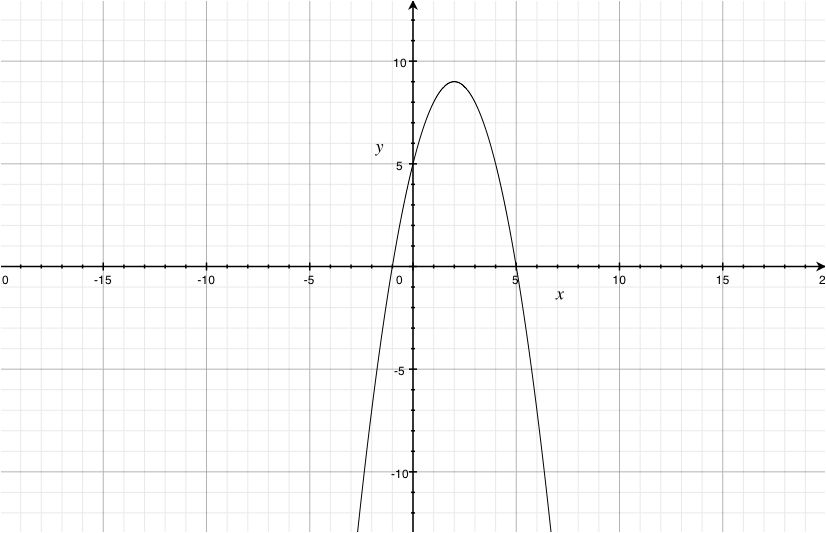Find the maximum/minimum point of the function y = x2 − 2x − 6
• Find the vertex using x = − [b/2a]x = − ( [( − 2)/2(1)] ) = 1y = ( 1 )2 − 2( 1 ) − 6 = − 7
• Determine which direction the parabola opens
a > 0 opens upwards
• Consider vertex and direction to determine maximum/minimum
(1, - 7) is a minimum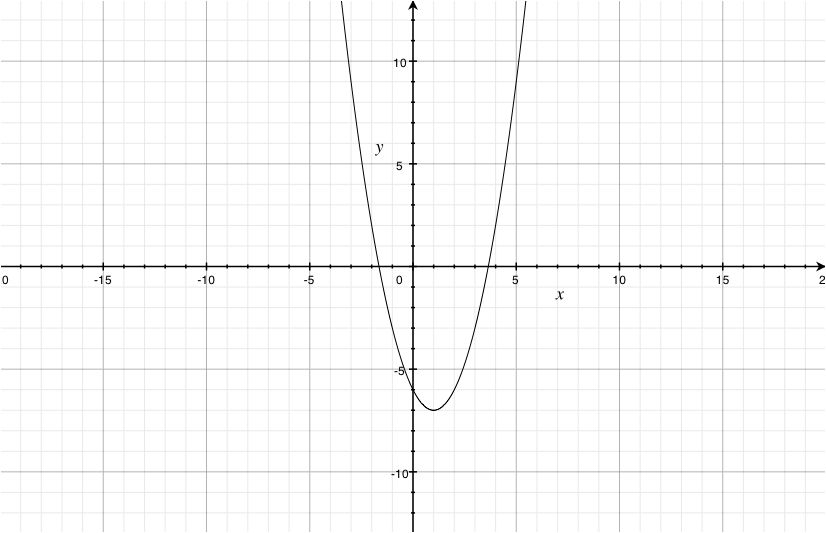Find the maximum/minimum point of the function y = [(x2)/4] + x
• Find the vertex using x = − [b/2a]x = − ( [1/(2([1/4]))] ) = − 2y = [(( − 2 )2)/4] + ( − 2 ) = − 1
• Determine which direction the parabola opens
a > 0 opens upwards
• Consider vertex and direction to determine maximum/minimum
( - 2, - 1) is a minimum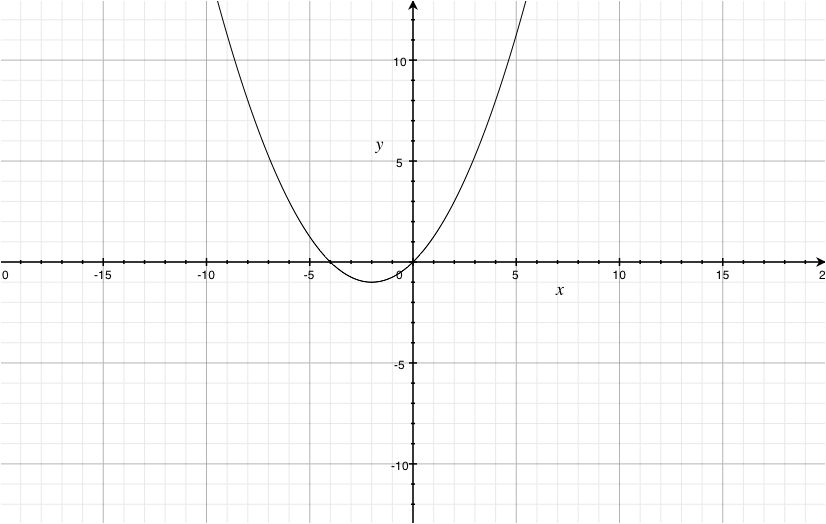Find the maximum/minimum point of the function y = − 5x2 + 10x + 4
• Find the vertex using x = − [b/2a]x = − ( [10/(2( − 5))] ) = 1y = − 5( 1 )2 + 10( 1 ) + 4 = 9
• Determine which direction the parabola opens
a < 0opens downwards
• Consider vertex and direction to determine maximum/minimum
(1,9) is a maximum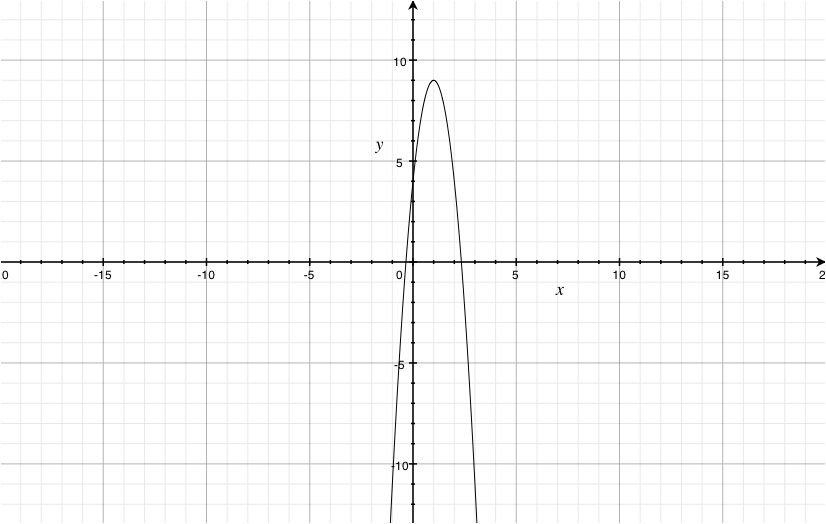Find the maximum/minimum point of the function y = [( − x2)/20] + 6
• Find the vertex using x = − [b/2a]x = − ( [0/(2( − [1/20]))] ) = 0y = [( − ( 0 )2)/20] + 6 = 6
• Determine which direction the parabola opens
a < 0opens downwards
• Consider vertex and direction to determine maximum/minimum
(0,6) is a maximum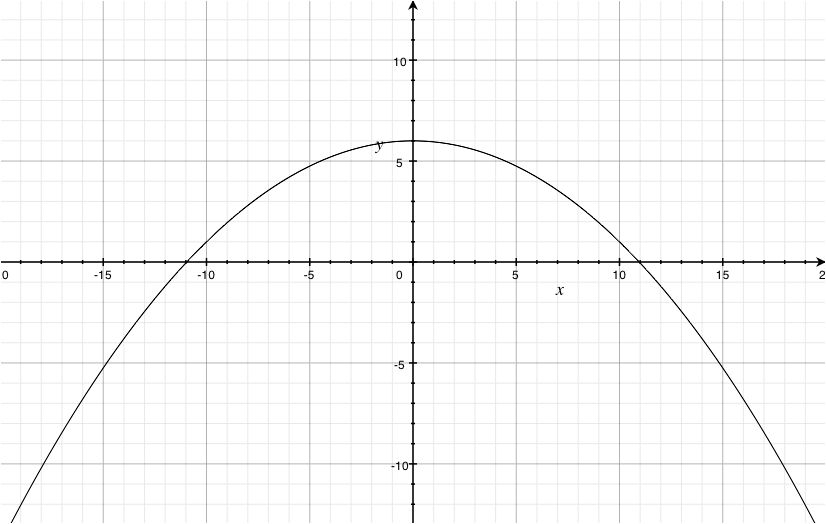Graph y = x2 + 4x + 4
• Find the vertex using x = − [b/2a]x = − ( [4/2(1)] ) = − 2y = ( − 2 )2 + 4( − 2 ) + 4 = 0
• Make a table of points
 x
 line
 − 2
 − 5
 − 4
 0
 1
 y
 line
 0
 9
 4
 4
 9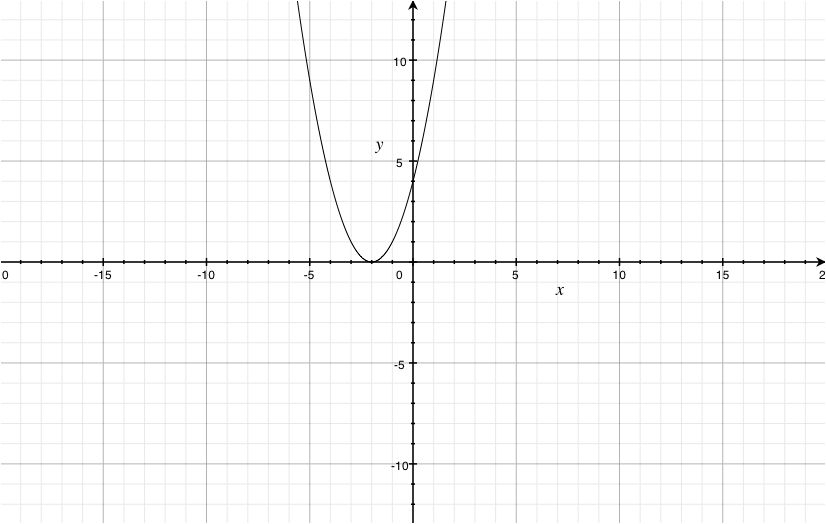Graph y = − x2 − 6x
• Find the vertex using x = − [b/2a]x = − ( [( − 6)/(2( − 1))] ) = − 3y = − ( − 3 )2 − 6( − 3 ) = 9
• Make a table of points
 x
 line
 − 5
 − 4
 − 3
 − 2
 − 1
 y
 line
 5
 8
 9
 8
 5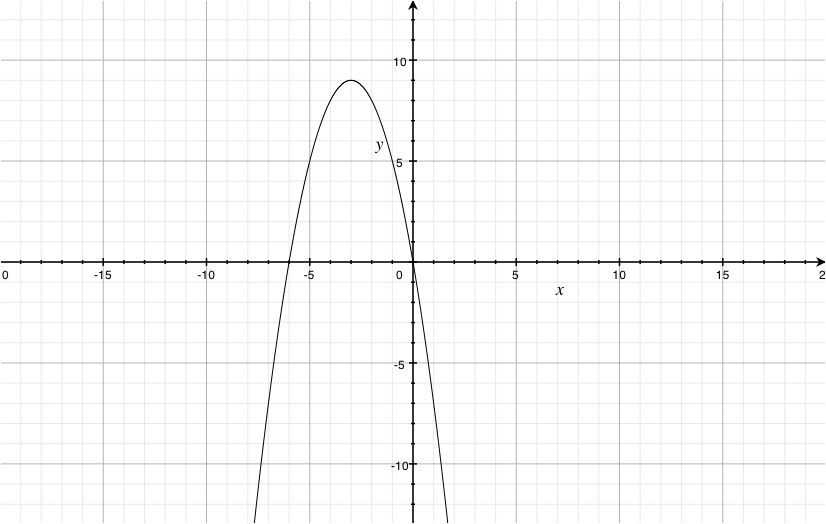Graph y = x2 − 10x + 18
• Find the vertex using x = − [b/2a]x = − ( [( − 10)/2(1)] ) = 5y = ( 5 )2 − 10( 5 ) + 18 = − 7
• Make a table of points
 x
 line
 3
 4
 5
 6
 7
 y
 line
 − 3
 − 6
 − 7
 − 6
 − 3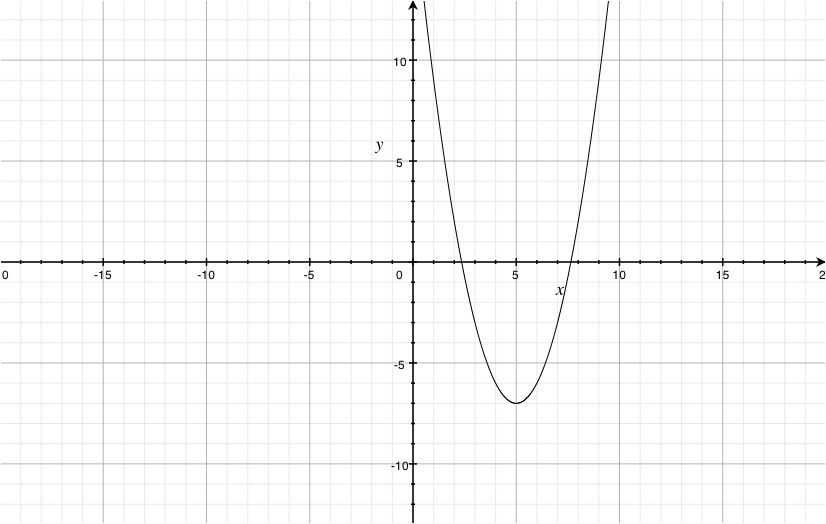Solve y = x2 + 4x utilizing axis of symmetry
• Find the vertex and line of symmetry using x = − [b/2a]x = − ( [4/2(1)] ) = − 2y = ( − 2 )2 + 4( − 2 ) = − 4
• Make a table of points
 x
 line
 − 2
 − 1
 0
 1
 2
 y
 line
 − 4
 − 3
 0
 5
 12
Graph to find x - intercepts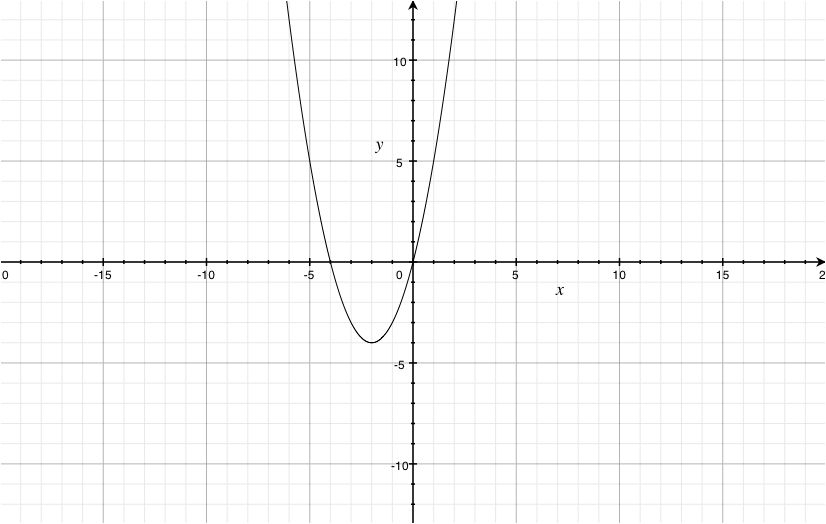x = − 4,0
Solve y = − x2 + 16x − 55 utilizing axis of symmetry
• Find the vertex and line of symmetry using x = − [b/2a]x = − ( [16/(2( − 1))] ) = 8y = − ( 8 )2 + 16( 8 ) − 55 = 9
• Make a table of points
 x
 line
 4
 5
 6
 7
 8
 y
 line
 − 7
 0
 5
 8
 9
Graph to find x - intercepts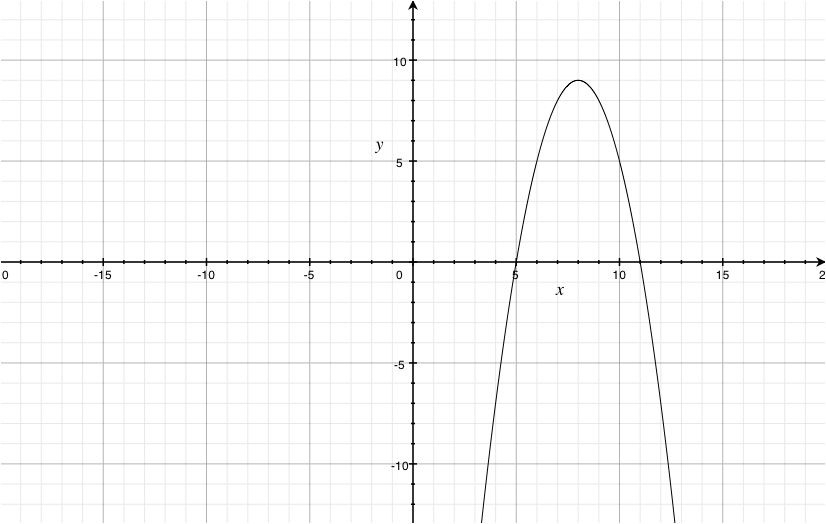x = 5,11

*These practice questions are only helpful when you work on them offline on a piece of paper and then use the solution steps function to check your answer.

Lecture Slides are screen-captured images of important points in the lecture. Students can download and print out these lecture slide images to do practice problems as well as take notes while watching the lecture.

• Intro 0:00
• Objectives 0:06
• Axis of Symmetry
• Vertex
• Transformations
• Graphing in Quadratic Standard Form
• Example 1 5:06
• Example 2 6:02
• Example 3 9:07
• Graphs of Quadratics Cont. 11:26
• Completing the Square
• Vertex Shortcut
• Example 4 13:49
• Example 5 17:25
• Example 6 20:07
• Example 7 23:43

Welcome back to www.educator.com.0000

In this lesson we are going to take a look at the graphs of quadratics.0002

We will first start off by looking at the basic shape of a quadratic and how it forms the shape known as the parabola.0009

We will pick up some new vocabulary such as the axis of symmetry and the vertex.0015

We will look at a few quadratics that are not written in standard form.0021

This will lead to a new technique that we have to use in order to apply out their graph.0025

We have seen this technique once before and this is known as completing the square,0031

but we will also use what is known as the vertex formula to do the same thing.0034

When you are looking at the shape of a quadratic it forms a parabola.0042

One way you can see this is it take the nice basic shape of x2 and plot out lots of different points on a graph.0048

Maybe choose some values like – 2, - 1, 0, 1 and 2, just to see what you get.0061

All of these guys are being squared.0067

-2 would give you 4, 12 would be 1, 02 would be 1 and 12 will be 1.0070

22 will be 4, and it will keep going in both directions.0077

Let us plot those out.0082

I’m not sure if you can see that it forms a nice parabola.0088

All other quadratics will have this parabola shaped to them.0097

Here are some features that you want to know about these parabolas.0103

One, they are only symmetric to align known as the axis of symmetry and runs right down the middle of that parabola.0107

Here are two examples that I have the axis of symmetry marked out.0116

Whatever that axis of symmetry intersects with the actual graph that point is known as the vertex0127

and you can see that on my little diagrams right here and right here.0134

Let us call those the vertex.0140

The reason why we want to know about the vertex is it will help us create a nice shortcut method for graphing these parabolas.0146

That way we would not necessarily have to go back to a large table of values every single time we want to graph them.0153

Think of this kind of in the context of when we are graphing out lines, when we are applying a whole bunch of points for those lines,0159

we simply had to find a y intercept and a slope.0165

With parabolas we do not have a notion of slope, but knowing where the vertex is can often help us plot out the rest of it.0169

We can use techniques known as transformations in order to figure out where the graph is.0181

This assumes that your quadratic is written in the following form of something like y= a × x - h2 + k.0187

I did not put in the function notation here but we could also imagine that being a y, either way will work out just fine.0196

If your quadratic is written in this very special form, there are lots of different things that it will tell you.0203

The (a) value out front will tell you whether it is been reflected over the x axis or if it had any type of vertical stretches.0210

If (a) is negative then your parabola will be facing down.0220

If it is positive your parabola will be facing up.0226

It is how we can also determine how wide or narrow to make that parabola.0231

If the absolute value of (a) is say greater than 1, then it will be a narrow parabola.0237

If the absolute value of a is less than 1, then you know it will be fairly wide.0248

It gives you a little bit about its shape.0259

The h and k are probably two of the most important things you can get rid of here and they help you determine where that vertex is.0262

h and k together would be the point of that vertex.0273

What h is helping you figure out is how far left or right the parabola ended up moving from its original spot said 0, 0.0277

The k helps you figure out how far up or down that parabola had to move from its original spot.0290

We will look at the h and k more as finding a vertex and then use the value of h to determine how wide or narrow it should be.0298

Let us see if we can identify some of these key things with the following problem.0310

First of all where is this axis of symmetry?0314

We will imagine a line straight down the middle of it, if we are to fold it up along this line it should match up with itself, axis of symmetry.0318

Now that we have that we can see where it intersects the graph.0335

We will say that this is the vertex.0341

We will go ahead and say what that location is.0345

It is that -4, 2.0347

Notice how this parabola is facing up, in the actual equation of it the (a) value should be positive.0350

Let us see if we can take a problem written in the special form and put it onto our graph.0364

I’m going to write down the general form, just so we can reference it.0372

It is y= ax - h2 + k.0376

The first thing you want to know is where is that vertex?0385

It is coming from looking at the h in the k.0388

Be very careful notice how in the formula here, it has a list of that as -h.0393

What we want to think of is if we had to rewrite this as x -,0401

what would that value need to be to make it match up with that 4 we have in the original.0407

It would have had to have been -4 and that will help us figure out the vertex a little bit better.0413

This tells us our vertex is at -4, 5.0421

Let us make that our first point on the graph.0432

-1, 2, 3, 4 and 1, 2, 3, 4, 5 right there0435

If we look at the (a) value out front, it is negative.0446

We know that our parabola should be facing down.0455

Do you know if it should be wide? Or should be narrow?0460

If we look at the absolute value of -2, we get 2 and that is greater than 1.0466

It is going to be more narrow than the standard parabola of y = x2.0473

Let us put this in comparison.0481

Suppose I were to graph a parabola facing down that was related to x2, that would be these points right here.0484

I want to write this light because that is not the parabola we want.0500

Our parabola is going to be narrower than this one.0503

In fact the values will be twice as much.0507

2, 3, 4, 5, 6, 7, 8.0514

Ours will look more like this, narrower than the normal x2.0521

A bit of a rough sketch we get an idea what is going on here.0530

If you want some more accurate things to put in here what you could do is plot out a few points or even just to add a few additional ones.0534

Let us try some more.0545

Let us see if we can work backwards by starting with the graph of a quadratic and see if we can make its equation.0549

Like before, one of the first important points we want to know is where is the location of that vertex?0557

That one is located at 4, 1.0563

Now that we have that I know what at least my h and k values are.0570

Y = ax -42 + 1.0577

It looks like the only thing I do not know right now is the value of a, and because the problems facing up, this should be a positive value.0586

It looks like it might be a little wider than our normal parabola of x2 so I'm thinking maybe it is a fraction of some sort.0600

To be able to figure this out, I’m going to take another point on the parabola that I know it is on there and substitute this value into my equation.0607

Then I will be able to solve that a directly.0615

Y is 2, x is 2.0618

You can see the only unknown we have in here is that a value.0627

2 - 4 would be -22.0633

Subtracting one from both sides 1 and -22 would give us 4.0640

We almost have that a value let us divide both sides by 4.0653

Now we can write down the entire equation.0665

This graph comes from ¼ (x - 42 + 1).0668

That vertex plays a key part in getting a connection between the graph and the equation.0679

Now, unfortunately, a lot of quadratic equations and functions are not written in that nice standard form.0688

In fact, we might end up with something that is written more like this.0696

When it is written like that, how are we supposed to figure out where that vertex is or which direction it is facing?0702

There are a couple of things you could do.0708

One is you directly take that and end up rewriting it into that nice standard form the a x - h2 + k + 4.0711

The way you accomplish that is you will go through the process of completing the square.0721

Once you have it in your nice standard form then you can go ahead and identify your vertex, which way it is going, is it going be wider.0727

You will know everything you need from there.0733

If you recall, completing the square can be quite a clunky process with lots of steps involved.0738

Fortunately, just like the quadratic formula, there is a way around.0745

Maybe not having to use completing a square and still plot out quadratics that look like this, like a x2 + bx + c.0751

You can package up a lot of these steps of completing the square and get what is known as your vertex formula.0760

It is this one right here.0768

It does look a little bit odd, but here is how you can interpret it.0770

This first value has given you a small formula so you can figure out the x coordinate of your vertex.0775

The second part of this, it has that –b/2a and what is that trying to tell you is that you should take the value you found for your x0784

You should evaluate it at that value.0795

One other thing to note, the vertex can sometimes be thought of as the minimum or the maximum of your quadratic.0801

Here it is a minimum point and here it is a maximum point.0812

We will use this vertex formula to help us out if our equation is written in this form.0818

Let us use completing the square to see how you could potentially use that as well to figure out what your vertex is.0832

In this one I have f(x) = 4x2 - 4x + 21 and in the method of completing the square first I need to organize things.0839

I will get my variables to one side.0848

I have f(x) and I’m going to subtract 21 from both sides.0851

That looks pretty good.0860

We need to make sure that the coefficient in front of x2 is 1.0862

Now it is 4, let us divide everything by 4, looking pretty good.0866

We need to go ahead and find the new number that we need to add to both sides to factor nicely.0886

We need to check our term in front of x in order to do that.0893

-1 ÷ 2 and then squared.0897

That would equal to ¼.0902

We need to add ¼ to both sides so that this will go ahead and factor.0904

On the right side there, it will factor nicely.0923

In fact this factors into x – ½2.0928

You can see that we are starting to build that similar form on the right side.0933

To see all of our other terms we need to go ahead and clean this up a bit and get that f(x) all by itself again.0942

I’m going to go ahead and combine the 21 and the 1 since they do have the same denominator down here.0952

That will be f(x) -20 ÷ 4.0960

Let us multiply both sides by 4.0972

That was quite a bit of work, but at least now we can see what is going on here.0993

These two values here, we can get rid of our vertex.0999

I'm at ½ , 20.1006

For my (a) value out here we can see that it should be facing up and the absolute value of 4 is larger than 1, it is going to be a narrow parabola.1013

Notice how we could have also figured out lots of information about our a value from the original equation out here.1026

This value here is the same a value as this one here.1034

You could get rid of that information at the very beginning.1040

Let us see if we can go ahead and graph another quadratic.1047

This one is f(x) = - x2 + 2x +5.1052

If we are going to graph this one, there are few things that we can do.1057

One, we could go through the process of completing the square, so we can figure out where its vertex is.1062

Or since it is in this form we might as well just use the vertex formula and get it directly.1066

That formula is –b ÷ 2a and once we find it we will put it back into the original problem.1076

Negative the value b is 2 ÷ 2 × a.1087

I have -2 ÷ -2 = 1.1094

This tells me that the x value of my vertex is 1.1099

We will borrow that and evaluate it back into the function.1105

What happens when we evaluate this at 1, -12 + (2 × 1) + 5.1108

12 is 1 that is -1 + 2 + 5 we have 6.1119

We have the location of the vertex.1130

It looks like it is at 1, 6.1131

What we need to know about a value to see which direction it is facing and looks like it is negative.1142

I know that this one is facing down.1151

Let us see what else we have figured out about it.1162

When it is written in this form, this last number will give us the y intercept.1164

I know it will go through 5.1170

Since the absolute value of our (a) term is exactly 1, then it is exactly as wide as our parabola x2.1175

Let us do that.1183

1, 1 will go out to 1, 2, 3, 4.1184

Now we can sketch out a nice graph of this parabola.1193

That vertex form can help out if you have your quadratic in this particular form.1200

Let us go ahead and sketch a graph of 1/3 x2 + 3x – 6.1209

This looks like another good one we should use our vertex formula on.1214

-b ÷ 2a then we will play it back in, -b ÷ 2a.1217

Negative our value of b is 3 ÷ 2 × -1/3.1229

This will be -3 ÷ -2/3.1238

Negative divided by negative will be a positive, we have 3 ÷ 2/3, which is the same as 3 × 3/2 = 9/2.1244

That will give me the x value of my vertex, something 9/2.1259

Now I have to take that and evaluate that by putting it back into the original function.1267

Take a little bit of work, so (-1/3 9/22) + (3 × 9/2) and then we will subtract 6.1272

9/22 will be 81/4 and I have 3 × 9/2 that will 27/2.1288

I can do a little bit of simplifying here.1305

3 goes into 81 27 times.1307

(-27 ÷ 4) + (27 ÷2) – 61311

That will to do a little bit of work if I'm going to get a common denominator.1319

I have to multiply by this fraction, top and bottom by 2 so 54/4.1325

We need a common denominator down here 24.1335

Slowly combining these together I have 27/4 - 24/4 = ¾ .1340

Quite a bit of work but now I know the location for sure of my vertex.1352

Let us see if we can now plot this out.1362

I need ½ , 2/2. 3/2, 4/2, 5/2, 6/2, 7/2, 8/2, and 9/2.1366

We will go up ¾1374

Not all the way up to 1 but just ¾ of that, right about here.1375

It looks like this particular parabola is facing down and because the 1/3 is going to be a fairly wide parabola.1382

How large that will make it?1398

I know it crosses through the y axis at -6.1400

We can put that value on there as well.1410

I have a good idea on what this looks like.1414

There is our sketch of the quadratic.1420

Let us do one last one just to make sure that we have all of these techniques down.1425

On this one, we want to find the maximum or the minimum value of our function.1429

This is just the same as finding the vertex.1434

We will go ahead and graph it out so you can see what that vertex is.1443

-b ÷ 2a, ½ (-b) ÷ 2a.1447

We will need both of those.1453

Starting off with trying to find the x coordinate.1456

Negative the value b2 ÷ 2 × a.1462

-2 ÷ - 4 will give us ½.1469

I would need to go ahead and put this back into the original.1478

-2 × ½2 + 2× ½ + 41485

A little bit of simplifying but not too bad -2 × ¼2 + 1 + 41493

-2 × ¼ = -1/2, 1 + 4 = 5 and now I can find a common denominator and put these two together.1509

I will get 9/2 for the second half of my vertex.1522

This vertex is located at ½, 9/2.1526

Let us put that on the graph.1533

½ for x and I have 1, 2, 3, 4, 5, 6, 7, 8, 9/2.1535

There is where our vertex is located.1542

The (a) value for this one is negative, so I know that the parabola is facing down.1545

It looks like it has a y intercept of 4.1553

It goes through that spot.1560

Be just a little bit more narrow than your average parabola because the absolute value of 2 is greater than 1.1563

Now that we sketched that out and from the sketch since it is facing down I can say that this vertex here is a maximum.1571

The maximum value of our function is that 9/2 that comes directly from the vertex which is located at ½ and 9/2.1584

A lot of different ways that you could go about actually sketching the graph of the quadratic and it is actually not so bad.1593

If it is in a nice standard form you can simply get rid of the vertex and its (a) value.1601

But if it is not in that form of then feel free to use the vertex formula.1606

Thank you for watching www.educator.com.1611

OR

### Start Learning Now

Our free lessons will get you started (Adobe Flash® required).